# Subtracting mixed numbers with like denominators | Fractions | Pre-Algebra | Khan Academy

##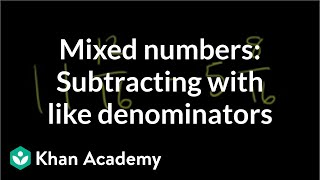By Khan Academy

subtract fractions with like denominators# One-step addition and subtraction equations with fractions and decimals

##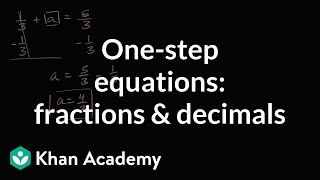By Khan Academy

Learn how to solve one-step addition and subtraction equations that have fractions and decimals in them.# One-step addition and subtraction equations with fractions and decimals

##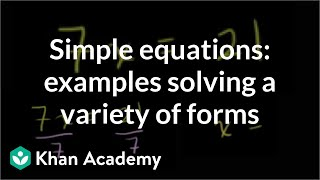By Khan Academy

Some quick examples to practice solving a variety of one step equations. All 4 operations (add, subtract, multiple, divide) are paired with variables.# One-step addition and subtraction equations with fractions and decimals

##By Khan Academy

Learn how to solve one-step addition and subtraction equations by adding or subtracting the same thing from both sides of the equation.# One-step addition and subtraction equations with fractions and decimals

##By Khan Academy

Learn how to solve this equation: a + 5 = 54ï¿½ï¿½ï¿½ï¿½ï¿½ï¿½ï¿½ï¿½ï¿½ï¿½ï¿½ï¿½# Addition and subtraction with significant figures | Decimals | Pre-Algebra | Khan Academy

##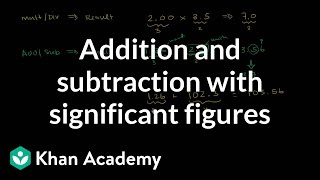By Khan Academy

This video shows us the rules for adding and subtracting measurements to show our level of accuracy. Note that the rules for addition/subtraction with significant figures are VERY different than the rules for mulitplication/division. Think about which number is the most precise!# Subtracting Mixed Numbers - YourTeacher.com - Pre Algebra Help

##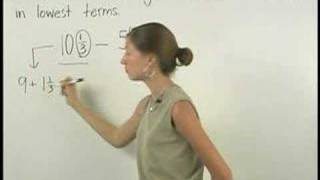By yourteachermathhelp

For a complete lesson on subtracting mixed numbers go to http://www.yourteacher.com - 1000+ online math lessons featuring a personal math teacher inside every lesson! In this lesson students learn to subtract mixed numbers by first subtracting the fractions then subtracting the whole numbers. For example to subtract 6 1/3 - 4 2/3 first subtract 1/3 ï¿½ 2/3. However notice that 1/3 ï¿½ 2/3 equals a negative fraction. In this situation the first fraction 6 1/3 can be rewritten as 5 + 1 1/3 or 5 + 4/3 or 5 4/3. Therefore the original problem 6 1/3 - 4 2/3 can be rewritten as 5 4/3 - 4 2/3. Now subtract the fractions 4/3 ï¿½ 2/3 to get 2/3 and subtract the whole numbers 5 ï¿½4 to get 1. So 5 4/3 - 4 2/3 = 1 2/3. Note that some of the problems in this lesson also require the student to find a common denominator for the fractions. For example 8 5/16 - 1 1/8.# Running distance in a week | Multiplication and division

##By Khan Academy

Solve a two-step word problem by drawing a picture and creating an equation.# Total seats in a theater | Multiplication and division

##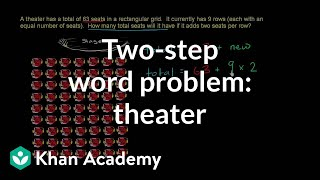By Khan Academy

Solve a two-step word problem by drawing a picture and creating an equation.# Marbles for friends | Multiplication and division

##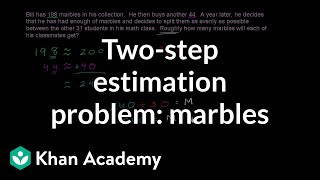By Khan Academy

Solve a two-step estimation word problem.# Algebra Basics: Solving Basic Equations Part 1 - Math Antics

##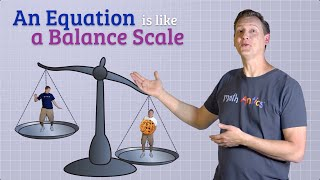By mathantics# Algebra Basics: Solving 2-Step Equations - Math Antics

##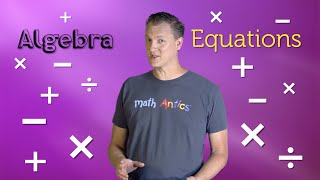By mathantics# Adding Rational Numbers

##By Anywhere Math# Algebra Basics: Solving Basic Equations Part 2 - Math Antics

##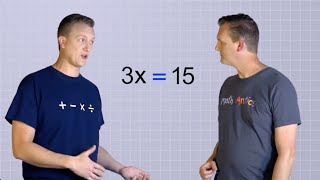By mathantics# Algebraic Expressions (Advanced)

##By Anywhere Math# ALL OF GRADE 9 MATH IN 60 MINUTES!!! (exam review part 1)

##By Lumos Learning

Here is a great exam review video reviewing all of the main concepts you would have learned in the MPM1D grade 9 academic math course. The video is divided in to 3 parts. This is part 1: Algebra. The main topics in this section are exponent laws, polynomials, distributive property, and solving first degree equations. Please watch part 2 and 3 for a review of linear relations and geometry. If you watch all 3 parts, you will have reviewed all of grade 9 math in 60 minutes. Enjoy! Visit jensenmath.ca for more videos and course materials.# The Arithmetic Series - Part 1 - 

##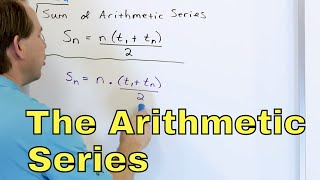By Math and Science# SAT Math Part 1 - Evaluating Functions and Solving Basic Equations - Algebra

##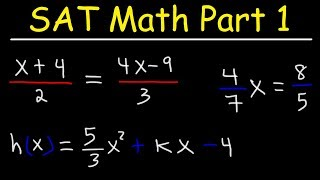By The Organic Chemistry Tutor# Algebra Basics: Laws Of Exponents - Math Antics

##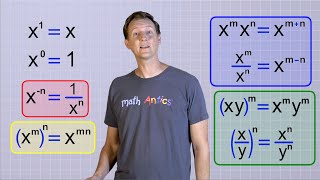By mathantics